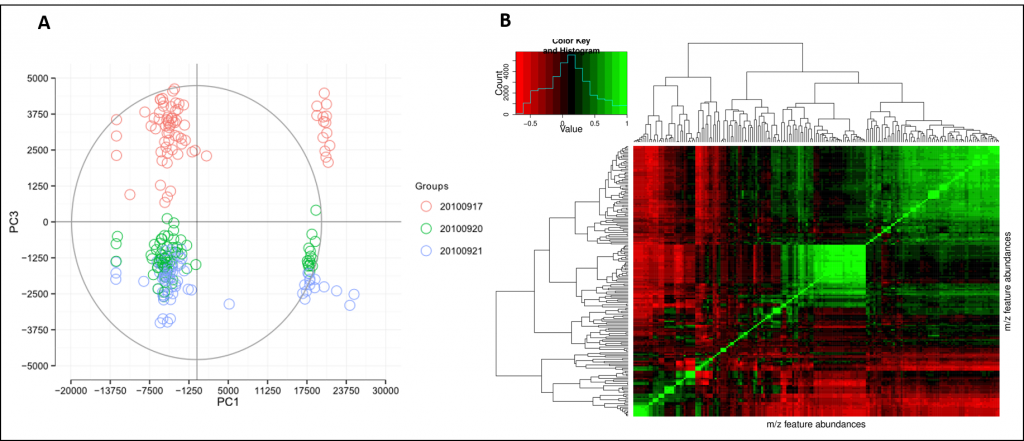# Analysis and interpretation

In the context of metabolomics, the most common statistical analysis approaches are grouped into univariate and multivariate methods. Each method offers unique insights into the data structure. Multivariate analysis works on a matrix of variables and highlights characteristics based on the relationships between all variables. Univariate analysis takes only one variable into account, resulting in differently weighted results.

The goal of statistical analysis is the categorisation and prediction of sample properties through generation of models that capture the information contained in data matrices. In mass spectrometry, the m/z ratio and signal intensity are the two most important variables. In NMR we select integrated signals of interest for data analysis.Figure 10 (a) Example PCA plot of three batches (red, green, blue). The red batch exhibits a strong batch effect. (b) Pearson’s correlation heatmap.

Without venturing too much into the area of statistics, principal component analysis (PCA, Figure 10a) and partial least squares (PLS) are established methods for multivariate analysis of metabolomics data. PCA is a method that enables us to reduce the dimensionality of our data into inferred variables, thus helping us to identify major trends and features.

The dimensionality-reduction methods can be used in classification, regression, and prediction exercises. The quality of the statistical models that we infer depends significantly on the data pre-processing, scaling and normalisation methods used. Therefore successful data analysis requires careful investigation of multiple models for consensus building (i.e. don’t rely on a single model!).

Figure 10b shows a correlation heatmap of a feature matrix, typically used in metabolomics analysis.